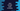# Java program to convert octal to binary## Write a program to convert octal to Binary in Java :

In this tutorial, we will learn how to convert one octal number to binary in Java. But before that, let’s check what is octal number and what is its relation with binary.

### Octal number System :

Octal number system is a base-8 number system, i.e. it uses numbers from 0 to 7. In decimal, we represent each number as base 10 . Similarly, in octal, each number is represented in base 8.

To convert a binary number to octal, start from right and take group of three digits of that number each time. Now, convert each three digits and that will be the octal representation for the number.

For example, let’s take example for 100. Binary representation for 100 is 1100100. Group each number : (1)(100)(100) . Convert each group of binary : (1)(4)(4). i.e. 144 is the octal representation of decimal 100. If we try to convert the octal to decimal = 1*(88) + 48 + 4*1 = 64 + 32 + 4 = 100

To convert a octal number to binary, we can simply convert each digit to binary format. That’s it.

### Program to convert Octal number to Binary :

``````/*
*
* you may not use this file except in compliance with the License.
* You may obtain a copy of the License at
*
*
* Unless required by applicable law or agreed to in writing, software
* WITHOUT WARRANTIES OR CONDITIONS OF ANY KIND, either express or implied.
* See the License for the specific language governing permissions and
*/

import java.util.ArrayList;
import java.util.Scanner;

/**
* Example Class
*/
public class ExampleClass {

/**
* System.out.println utility method
*
* @param value : value to print
*/
static void print(String value) {
System.out.println(value);
}

/**
* Method to convert a decimal number to Binary
*
* @param no : Decimal number
* @return : Binary number in String form
*/
static String decimalToBinary(int no) {
String binaryResult = "";

while (no > 0) {
binaryResult = (no % 2) + binaryResult;
no = no / 2;
}

return binaryResult;
}

/**
* Method to convert octal number binary
*
* @param octal : Octal number
* @return : Binary number in string format
*/
static String octalToBinary(int octal) {
String octalResult = "";
int reminder;
String binary;

while (octal != 0) {
//pick each digits of this number and convert it to binary
reminder = octal % 10;

//convert it to binary
binary = decimalToBinary(reminder);

if (octal > 8) {
//binary number is of length 3. If not, add extra zeros to front . Don't add for the last digit , i.e
// for the last iteration
if (binary.length() == 1) {
binary = "00" + binary;
} else if (binary.length() == 2) {
binary = "0" + binary;
}
}
//append the binary format to the result string
octalResult = binary + octalResult;

//reduce the value. If previously it was 332, next time it will be 33
octal = octal / 10;
}

//return the result
return octalResult;
}

/**
* main method for this class
*/
public static void main(String[] args) {

int octalNum;

Scanner scanner = new Scanner(System.in);

print("Enter a Octal Number : ");

octalNum = scanner.nextInt();

print("Binary representation of " + octalNum + " is : " + octalToBinary(octalNum));

}
}
``````

### How it works :

1. Main algorithm of the above program is to take each digit of a number and convert it to a three digit binary number. Append these binary numbers and that will be the output.

2. We have two methods here : first one is to convert a octal number to binary : the main method will call it first.

3. Next we will pick each digit of the given number . e.g. if the input number is 234, first we will take 4, then 3 and after that 2.

4. Each number picked will be converted to its three digit binary representation. For the above example, we will first convert 4 i.e. ‘100’ , then 3 i.e. ‘011’ and finally 2 i.e. ‘010’. But for the last number, i.e. first digit, don’t add any starting ‘0’. So, 2 will be ‘10’.

5. Finally append all binary representations and return the result as a String.

### Sample Output :

``````Enter a Octal Number :
112
Binary representation of 112 is : 1001010``````
``````Enter a Octal Number :
7
Binary representation of 7 is : 111``````